• MATLAB求解变量方程的方法，solve命令用来求解方程
• 优化设计-单变量函数求极值-黄金分割法与二次差值法-MATLAB编程求解 目录优化设计-单变量函数求极值-黄金分割法与二次差值法-MATLAB编程求解黄金分割法二次插值法原函数图像 以前做过的优化设计作业，现将MATLAB程序...
优化设计-单变量函数求极值-黄金分割法与二次差值法-MATLAB编程求解
目录优化设计-单变量函数求极值-黄金分割法与二次差值法-MATLAB编程求解黄金分割法二次插值法原函数图像
以前做过的优化设计作业，现将MATLAB程序与运算结果附在下方，分享出来欢迎大家指点！
两种方法求同一函数，相同区间，相同精度的极值点。
黄金分割法
黄金分割法计算流程图比较简单，现将题目、程序、运算结果附在下方：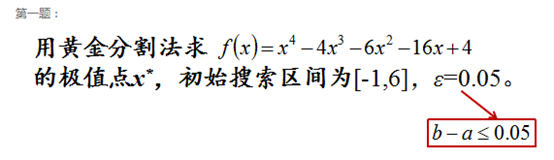MATLAB程序如下：
%% 一维搜索法求函数极值
clear all;clc
%%%%%%%%%%%%%初始参数设定%%%%%%%%%%%%%%%%
f=@(x)x^4-4*x^3-6*x^2-16*x+4;
area=[-1,6];%区间确定
a=area(1);
b=area(2);
r=(sqrt(5)-1)/2;%定义区间缩小比例
e=0.05;%精度确定
d=b-a;
i=0;
%%%%%%%%%%作图用于验证结果是否正确%%%%%%%%
x=a:0.01:b;
y=x.^4-4*x.^3-6*x.^2-16*x+4;
plot(x,y)
%%%%%%%%%%%%%循环计算语句%%%%%%%%%%%%%%%%
while d>e
a1=b-r*d;
b1=a+r*d;
f1=feval(f,a1);
f2=feval(f,b1);
i=i+1;
A(i)=a;
B(i)=b;
if f1<f2
b=b1;
else a=a1;
end
d=b-a; %精度计算
end
%%%%输出极值点与每次运算得到的a,b的值%%%%%%
X(1)=(b+a)/2
A=A';B=B';
[A]
[B]


此程序并未完全按照黄金分割法流程进行，即每次进行a1,b1点计算过程中并未利用上一次计算结果，每次循环将多一次运算，但优势在于程序在初始参数设定时设定了区间单次缩小比例[r]，使得程序对于任意缩小比例的一维搜索法皆可使用。
若想要程序运行完全满足黄金分割法要求，即每次计算a1或者b1时可利用上一次计算过程中的数据，则在程序循环判断中再一次细化运行即可，但此时程勋运算也多一次判断过程，个人认为在数值并不巨大或者复杂的情况下，程序速度无太大提升。
程序结果如下，同时记录了每次迭代时区间的端点值a,b，最终得到极小值点X=3.9968，迭代计算次数为：11次，取值表如下：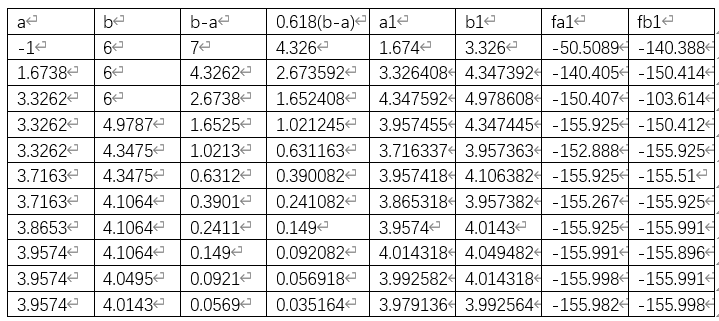二次插值法
题目、程序、运算结果如下：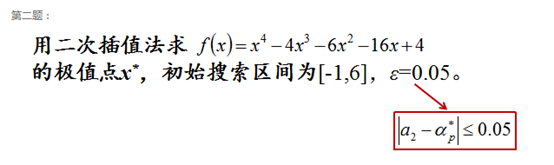MATLAB程序如下：
%二次插值法求函数极值
clear all;clc
%%%%%%%%%%%%%初始参数设定%%%%%%%%%%%%%%%%
f=@(x)x^4-4*x^3-6*x^2-16*x+4;
area=[-1,6];%%%%区间确定%%%%
a1=area(1);
a3=area(2);
a2=(a1+a3)/2;%%%%a1<a2<a3%%%%%
e=0.05;%%精度确定%%
d=a3-a1;
i=0;
%%%%%%%%%%%%%循环计算语句%%%%%%%%%%%%%%%%
while d>e
f1=feval(f,a1);
f2=feval(f,a2);
f3=feval(f,a3);
c=(a2-a3)*f1+(a3-a1)*f2+(a1-a2)*f3;
ap=0.5*((a2^2-a3^2)*f1+(a3^2-a1^2)*f2+(a1^2-a2^2)*f3)/c;
fp=feval(f,ap); %%%%求取ap,fap%%%%
i=i+1;
A(i)=a1;
B(i)=a2;
C(i)=a3;
if ap>a2
if f2>=fp
d=abs(a2-ap);
a1=a2;
a2=ap
else
d=abs(a2-ap);
a3=ap
end
else
if f2>=fp
d=abs(a2-ap);
a3=a2;
a2=ap;
else
d=abs(a2-ap);
a1=ap;
end
end
end
%%%%输出极值点与每次运算得到的a,b，c的值%%%%%%
X(1)=ap
A=A';B=B';C=C'
[A]
[B]
[C]


程序结果如下，同时记录了每次迭代时所取的a,b,c的值，最终得到极小值点X=3.9501，迭代计算次数为：7次，取值表如下：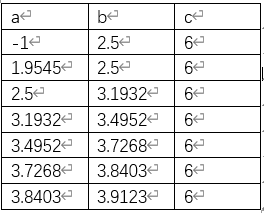原函数图像
得到两种不同方法的计算结果后，通过MATLAB生成原函数的图像用于验证程序运行的准确性，并比较二者优缺点。原函数图像如下：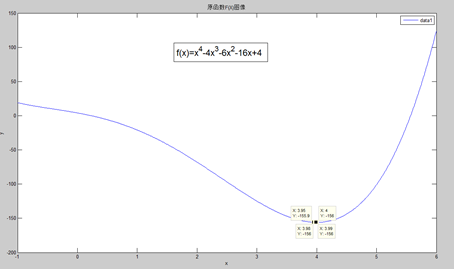放大极小值点处图像：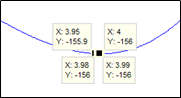由图可知两种求取极值的方法都能较好的逼近函数极值点，在本题程序中，同精度下，黄金分割法所求取的极值点更为逼近原函数实际极值点，但迭代计算次数更多为11次，而二次插值法求取的极值点虽不如黄金分割法求取的极值点准确，但迭代计算次数更少，为7次。二者各有优缺点，可根据实际需要选取方法进行问题求解。


展开全文• 求解步骤： （1）先定义符号变量 （2）列出来方程组 （3）替换下式中的量syms a b c d e vm r Y Nm; % Y = a*vm + b*e + c*r ; % Nm = d*r + c*( vm+e ); [vm,r] = solve('Y = a*vm + b*e + c*r ','Nm = d*r + c*...
求解步骤：
（1）先定义符号变量
（2）列出来方程组
（3）替换下式中的量

syms a b c d e vm r Y Nm;
%  Y = a*vm + b*e + c*r ;
%  Nm = d*r + c*( vm+e );
[vm,r] = solve('Y = a*vm + b*e + c*r ','Nm = d*r + c*( vm+e )','vm','r')

备忘一下
展开全文• 利用matlab编程求解人口预测模型.doc利用matlab编程求解人口预测模型一、名词和符号说明名词解释：(1)拟合: 对于某个变化过程中的多个相互依赖的变量，可建立适当的数学模型，用于分析预报决策或控制该过程....
利用matlab编程求解人口预测模型.doc利用matlab编程求解人口预测模型一、名词和符号说明名词解释：(1)拟合: 对于某个变化过程中的多个相互依赖的变量，可建立适当的数学模型，用于分析预报决策或控制该过程.对于两个变量可通过用一个一元函数去模拟这两个变量的取值.用不同的方法可得到不同的模拟函数.下面使用图表介用Mathematica做曲线拟合。(2)差分方程:含有自变量，未知函数以及未知函数差分的函数方程，称为差分方程。(3)迭代法:是牛顿在17世纪提出的一种求解方程f(x)=0.多数方程不存在求根公式，从而求精确根非常困难，甚至不可能，从而寻找方程的近似根就显得特别重要。 设r是f(x)=0的根，选取x0作为r初始近似值，过点(,f())做曲线y=f(x)的切线L，L的方程为,求出L与x轴交点的横坐标 ,称为r的一次近似值，过点(,f())做曲线y=f(x)的切线，并求该切线与x轴的横坐标称为r的二次近似值，重复以上过程，得r的近似值序列{Xn},其中,称为r的n+1次近似值。上式称为牛顿迭代公式。符号说明：第 k年i岁的女性总人数女性人口的(按年龄)分布向量第k年i岁的女性生育率第k年i岁的女性死亡率第 k年i岁的女性存活率i岁女性的生育模式          k年总和生育率(控制人口数量的主要参数)A            存活率矩阵B            生育模式矩阵二、模型假设针对本题中出现的数据的代表意义和建立模型时能够使问题理想化、简单化，我们应用已知数据，将其时间离散化,由于女性是影响总人口变化的主要因素 ,因此本模型从考虑女性人口的发展变化出发， 我们在不失科学性的前提下作出如下合理的基本假设：假设女性最大年龄为90岁,最小年龄为0岁，以1岁为1个年龄组,1年为1个时段，不考虑同一时间间隔内人口数量的变化。(2) 中短期内，总和生育率、死亡率和出生性别比不会发生大的波动，可以以往年平均值代替预测值；(3) 长期人口预测的参数主要由政策决定；(4) 死亡率只与年龄有关，不考虑生存空间等自然资源的制约,不考虑意外灾难等因素对人口变化的影响。(5) 生育率仅与年龄和时间有关,存活率也仅与年龄有关。(6) 育龄区间为[14，49]。(7) 在讨论乡村人口城镇化时，只考虑乡到城镇的迁入与迁出。(8) 流入流出人口不改变该地区的人口性别、年龄结构。三、模型的建立与求解求解预测中长期人口增长问题首先我们来建立一个离散的人口增长模型, 由于女性是影响总人口变化的主要因素 (考虑性别比即可得到总人口数量)所以我们借助于女性人口的发展变化规律来分析和预测总人口的发展变化趋势。引入Leslie人口模型,利用差分方程，既可得到离散型的人口模型。a 、问题分析根据附录2已有的数据，分别针对市、镇、乡人口的不同情况建立三个差分方程模型，运用Matlab 求解，再用Excel软件描绘出人口数量变化的趋势，对中国人口数量增长做出中短期(10—20年)和长期(50年以后)的分析和预测，确定人口增长的总趋势，并依据《中国人口统计年鉴》中已有人口总数进行模型验证。b 、模型建立首先，参照附表中的数据，由于市、镇、乡差距较大，我们将分别进行研究。:第k年i岁的女性生育率;     : k年总和生育率，或生育胎次;:第k年i岁的女性死亡率;         :第 k年i岁的女性存活率: i岁女性的生育模式，用表示女性人口的(按年龄)分布向量,记A=B=则模型应表示为：=A+B利用matlab软件编程求解，程序如下：c=zeros(91);d1=[   … … ];for i=1:91for j=1:91if i==jc(i+1,j)=d1(i)endendendA=c1a1=[  … … ];b=zeros(91);for i=1:35b(1,i+15)=a1(i)endB=b1;=[  … …]        %2001对应初始值y=zeros(91,n)%n表示要预测年数y(:,1)= ；for k=1:19y(:,k+1)=A*y(:,k)+(k)*B*y(:,k)end(一)用此模型预测中短期女性人口变化趋势考虑到男女性别比例波动不大，所以女性人口数量的发展趋势可以预测全国总人口的发展趋势。对所给数据进行处理，发现近期(k)变化很小，这里我们取=/5即：市：=1；镇：=1.254；乡：=1.649，代入模型方程，得：x(k)=……………………………………………………………(3
展开全文• 1.简介在Matlab中，fmincon函数可以求解带约束的非线性多变量函数(Constrained nonlinear multivariable function)的最小值,即可以用来求解非线性规划问题matlab中，非线性规划模型的写法如下2.基本语法[x,fval]=...


1.简介在Matlab中，fmincon函数可以求解带约束的非线性多变量函数(Constrained nonlinear multivariable function)的最小值,即可以用来求解非线性规划问题matlab中，非线性规划模型的写法如下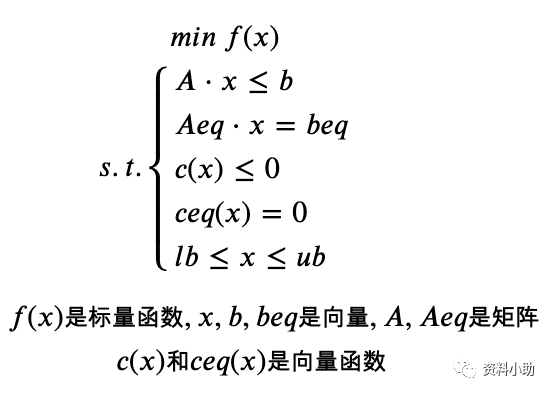2.基本语法[x,fval]=fmincon(fun,x0,A,b,Aeq,beq,lb,ub,nonlcon,options)x的返回值是决策向量x的取值，fval的返回值是目标函数f(x)的取值fun是用M文件定义的函数f(x),代表了(非)线性目标函数x0是x的初始值A,b,Aeq,beq定义了线性约束 ,如果没有线性约束，则A=[],b=[],Aeq=[],beq=[]lb和ub是变量x的下界和上界，如果下界和上界没有约束，则lb=[],ub=[],也可以写成lb的各分量都为-inf,ub的各分量都为infnonlcon是用M文件定义的非线性向量函数约束options定义了优化参数，不填写表示使用Matlab默认的参数设置3.实例示例,求下列非线性规划：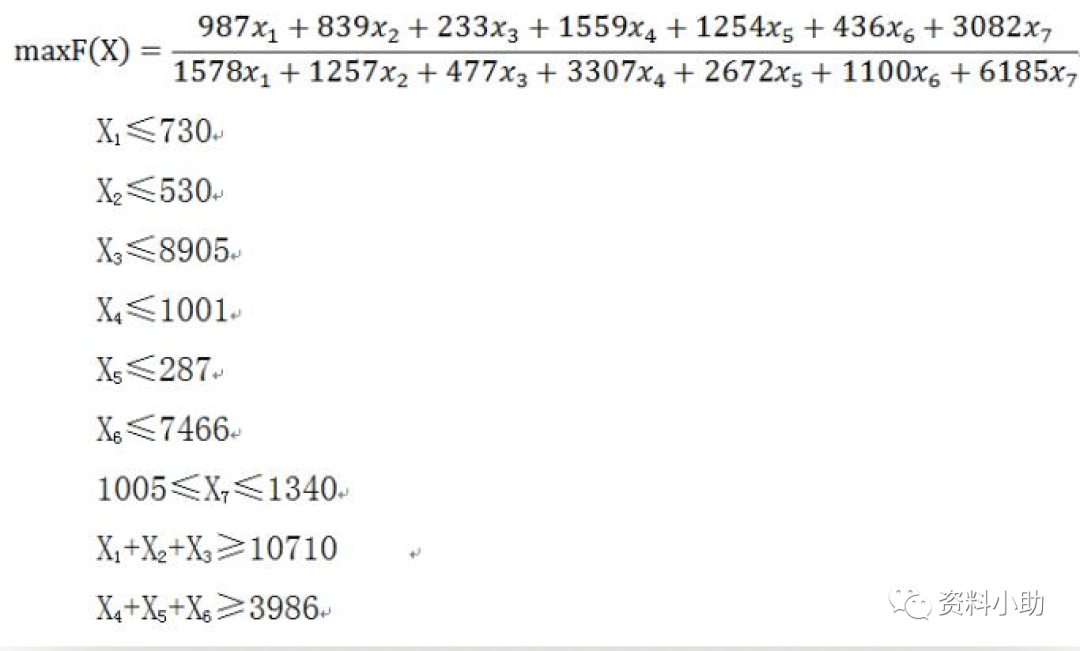首先，编写目标函数的M函数文件，由于求得是最大值，所以先化为求最小值问题，在原目标函数前面添加负号即可，M函数文件如下，保存为fun.m.function f = fun(x)f = -(987*x(1)+839*x(2)+233*x(3)+1559*x(4)+1254*x(5)+436*x(6)+3082*x(7))/(1578*x(1)+1257*x(2)+477*x(3)+3307*x(4)+2672*x(5)+1100*x(6)+6185*x(7));end然后，编写线性和非线性约束不等式已经非线性约束等式的M函数文件，保存为nonlinearcondition.m，代码如下function [f,ceq]= nonlinearcondition(x)f(1) =x(1) - 730;f(2) =x(2) - 530;f(3) =x(3) - 8950;f(4) =x(4)-1001;f(5)=x(5)-287;f(6)=x(6)-7466;f(7)=x(7)-1340;f(8)=1005-x(7);f(9)=-x(1)-x(2)-x(3)+10710;f(10)=-x(4)-x(5)-x(6)+3986;ceq = 0;%由于没有非线性约束等式，所以这一项写 0end最后，在Command窗口输入如下代码[x,fval] =fmincon('fun',[0;0;0;0;0;0;1100],[],[],[],[],[0;0;0;0;0;0;1100],[],'nonlinearcondition')即可得到最优解和最优值，最优值分别为x =   1.0e+03 *    0.8550    0.6550    9.0750    0.5374    0.1452    3.6801    1.2649fval = -0.4884         目标函数最优值为z = -fval=0.4884.

展开全文• matlab求解变量函数极限，高阶导数解析解。含源码，图例，一并合在word里，office2007.所有源码都已实际测试，版本MATLAB7.9.0
• ## 如何利用matlab求解方程

万次阅读 多人点赞 2018-04-22 12:46:54
如何利用matlab求解方程1. 前言作为三大数学软件之一，matlab在数值计算方法的能力首屈一指。...2. 用法求解过程2.1 指明变量告诉电脑方程中所含有的变量，包括参数和未知变量。比如：所求解的方程为：，很显然...
• 最优化问题的求解;若要计算一个函数 f (x) 的最大值可根据f (x) 的最大值= - f (x) 的最小值得到 目前所有的最优化算法均不能保证求出全局最优解即整个定义域上的最值以下介绍的命令实际上是求极小值局部最小
• 1 % 符号变量 两种表达方式 2 a=sym('a'); 3 class(a); 4 5 syms b; 6 b; 7 8 % 符号常量 9 c=sym('3'); 10 c; 11 % 符号表达式 三种表达方式 12 f1='3*x+4'; % char类型，但是可以参与运算。...
• Matlab求解线性规划问题——linprog函数： 引用博客链接侵删 [x,fval,exitflag,output,lambda] = linprog(c,A,b,Aeq,beq,LB,UB,X0,OPTIONS) 其中，fval返回目标函数的值，exitflag是收敛标志，取1则问题收敛，output...
• 6.1 代数方程 solve (f,t) 功能对变量t 解方程f=0t 缺省时默认为x 或最接近字母x 的符号变量求解一元二次方程f=a*x^2+b*x+c的实根 syms a b c x f=a*x^2+b*x+c; solve (f,x) ans= [1/2/a(-b+
• 可能是版本的问题，我的2013a可以运行result =[ 0.667, 0.8]result =[ 1.0, 1.0]result =[ 1.33, 1.14]result =[3.41, -2.19][ 0.586,1.04]result =[ 1.0,1.0][ 3.0, -1.5]result =[ 2.0, 0]result =[ 0.539 - 8.73e...
• 1.简介在Matlab中，fmincon函数可以求解带约束的非线性多变量函数(Constrained nonlinear multivariable function)的最小值,即可以用来求解非线性规划问题matlab中，非线性规划模型的写法如下2.基本语法[x,fval]=...
• % 求解 for i=1:N; % 运算次数 p=subs(f,{u ,v },{x0(1) x0(2)});% 为subs函数的赋值运算的矩阵 q=subs(df,{u ,v },{x0(1) x0(2) });% 为subs函数的赋值运算 2*2的矩阵 x =x0 - grass(q,p);% 得到的一组新数值...
• 粒子群算法matlab求解函数最优解为例 clear; clc; close all; N=100; %粒子个数 D=2; %粒子维数 MaxIter=500; %最大迭代次数 C1max=1.8; %权重参数，自适应 C2max=1.8; C1=1.2; C2=1.2; w=0.79; Wmax=0.8; %对...数学建模 算法
• LINGO的语法规定 1求目标函数的最大值或最小值分别用MAX=或MIN=来表示 2每个语句必须以分号结束每行可以有许多语句语句可以跨行 3变量名称必须以字母(A~Z)开头由字母数字(0~9)和下划线所组成长度不超过32个字符不...
• 这里面涉及到了两个难点：（本人matlab小白一枚，高手勿喷．） １．求解定积分 ２．已知函数因变量的值，求自变量的值 解题思路如下： 一　求解F(q)对应的q值 尝试１：求出F(q)的值为0.3758--&gt;find(y1==...
• 优化类问题相对要难一些，主要就难在模型求解部分，本文来给大家介绍一些MATLAB求解数学规划模型的案例。1、如何建立优化模型？为了更直接地说明问题，我们先来看几个例子：问题一：某工厂在计划期内要安排生产 I、...
• 一篇论文，关于MATLAB环境下神经网络求解变量问题的电子书。侵删。 摘要如下:在科学工程领域， 经常存在因变量与自变量关系不确定的情况。 如果因变量与自变量的关系式的类型是已知的，则可以利用相应的回归方法...
• limit(F,a)：符号表达式F采用默认自变量（可由函数findsym求得），该函数求F的自变量趋于a时的极限值。 limit(F)：符号表达式采用默认变量，并以a=0作为自变量的趋近值。 limit(F,x,a,'right')或limit(F,x,a,'...
• 使用方法： roots(num) 其中，num是方程的系数数组，并且按照自变量幂次降序排列 示例： 求解方程3x^2-2x-4=0的根 >> roots([3 -2 -4]) ans = 1.5352 -0.8685
• ConstrainedMPC.m 滚动求解，生成最优控制量。 理论部分见https://blog.csdn.net/m0_37764065/article/details/107207726 function [k,r,y1,u1,x1,x2,x3,yout] = ConstrainedMPC(Ad,Bd,Cd,Nc,Np,rw,r,u_min,u_max...
• MATLAB里solve、fzero、fsolve、root等函数用于求解方程（组） 1.solve函数 主要用于求解代数方程和非线性方程 [x1,……]=solve（‘F’，……，‘var’，……） F表示方程，var表示求解变量。 x1表示求解结果。 ...
• Matlab求积分@TOC 一些最近学习的总结，希望可以帮助到，不对的地方欢迎批评指正 首先是求定积分： 方法一： 利用intergral 数值积分（用于求定积分）：在计算时要用到索引@，@可以理解为，加了这个东西，他后边跟的...算法
• ## Matlab学习——求解微分方程(组)

万次阅读 多人点赞 2018-05-29 17:18:58
1.在 Matlab 中，用大写字母 D 表示导数，Dy 表示 y 关于自变量的一阶导数，D2y 表示 y 关于自变量的二阶导数，依此类推.函数 dsolve 用来解决常微分方程（组）的求解问题，调用格式为 X=dsolve(‘eqn1’,’eqn2’...
• 定义符号变量 调用dsolve函数 [y1,...,yN]=dsolve(eqns,conds,Name,Value) eqns为符号微分方程或符号微分方程组；conds为初值条件或边界条件；Name和Value为可选的成对参数。 求 x2+y+(x−2y)y′=0 x^2+y+\left( x...
• ## Matlab求解线性规划

千次阅读 2019-09-14 21:06:57
MATLAB是一种强大的数学工具软件，以下将介绍线性规划模型的MATLAB求解。 1. 线性规划的标准型 目标函数： 约束条件： 其中，是目标函数的系数矩阵，称为决策变量， 是不等约束系数矩阵，是不等约束常数......

# matlab变量求解matlab 订阅